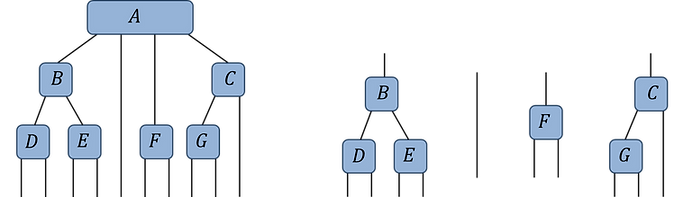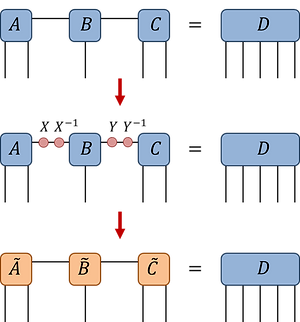##### Tutorial 3: Gauge Freedom

In this tutorial you will learn about manipulating the gauge freedom in tensor networks, and how this freedom can be exploited in order to achieve an optimal decomposition of a tensor within a network. Topics include:

• Tree tensor networks

• Gauge freedom in tensor networks

• Shifting the center of orthogonality

• Tensor decompositions within networks

###### Python

The box to the right shows the code set-up necessary to run the example snippets given throughout this tutorial:

##### T3.1: Tree tensor networks

In this tutorial we shall focus only on tensors networks that do not possess closed loops (i.e. they are described by acyclic graphs). This class of tensor network, which we generically refer to as tree tensor networkspossess many nice properties that networks containing closed loops lack and are thus much easier to manipulate. However, most of the results presented in this tutorial regarding gauge freedom can be generalized to the case of networks containing closed loops, as discussed in this reference

Fig.3.1(a) presents an example of a tree tensor network. If we select a tensor to act as the center (or root node) then it is always possible to understand the tree tensor network as being composed of a set of distinct branches extending from this chosen tensor. For instance, Fig.3.1(b) depicts the four branches (including one trivial branch) extending from the order-4 tensor A from Fig.3.1(a). Importantly, connections between the different branches are not possible in networks without closed loops.

###### Fig.3.1(b): Branches w.r.t. tensor 'A'##### T3.2: Gauge freedom

Let T be a tensor network that, under contraction of all internal indices, evaluates to some tensor D. In this tutorial we shall concern ourselves with the uniqueness of the decomposition: is there a different choice of tensors within the network that will still evaluate to the same tensor D?

Clearly the answer is yes! As shown below in Fig.3.2(a-b), on any internal index of the network one can introduce a resolution of the identity (i.e. a pair of matrices X and X^(-1)) which, by construction, does not change the final product that the network evaluates to. However absorbing one of these matrices into each adjoining tensor does change their content (while leaving the geometry of the network unchanged). Thus we conclude that there are infinitely many choices of tensors such that the network product evaluates to some fixed output tensor. We refer to this ability to introduce an arbitrary resolution of the identity on an internal index as the gauge freedom of the network.

###### Fig.3.2(a): Gauge change###### Fig.3.2(b): RedefinitionsWhile in some respects the gauge freedom is a nuisance (as it implies tensor decompositions are never unique), it can also be exploited to simplify many types of operations on tensor networks. Indeed, most tensor network algorithms require fixing the gauge in a prescribed manner in order to function correctly. We now discuss several ways to fix the gauge degree of freedom in such a way as to create a center of orthogonality, and the utility of doing so.

##### T3.3: Creating a center of orthogonality

Def.3.3: Center of Orthogonality

Let T:{A,B,C,…} be a tree tensor network, then a tensor A is a center of orthogonality if, for every branch of the network attached to A, the branch forms an isometry between its open indices and the index connected to tensor A.

###### Fig.3.3(a): Tensor network T###### Fig.3.3(b): Isometric branch constraintsIn the example above, the tensor A from the network T in Fig.3.3(a) is a center of orthogonality if and only if the constraints of Fig.3.3(b) are satisfied, which demand that each of the branches connected to A forms an isometry. Here, as with Tutorial 2, the conjugate Bof a tensor B denotes complex conjugation as well as opposite vertical orientation in figures.

We now discuss two different methods for changing the gauge in network T to make any tensor A into center of orthogonality, before later revealing the significance of doing so.

Setting a center of orthogonality method 1: ‘Pulling Through’

Here we describe a method for setting a tensor A within a network T as a center of orthogonality through iterative use of the QR decomposition. (Alternatively one can use the SVD to achieve the same effect, although the QR decomposition is usually preferred as it is computationally quicker). The idea behind his method is very simple: if we transform every individual tensor within a branch into a (properly oriented) isometry, then the entire branch collectively becomes an isometry and thus satisfies the requirement of Def.3.3.

(i) Using the network of Fig.3.3(a) as an example, begin by orienting each index with an arrow that points towards the chosen center tensor A

(ii) Then, starting from a tensor at the tip of a branch, perform a QR decomposition on the tensor (under the partition between incoming and outgoing arrows). Next redefine the tensor in question as the orthogonal ‘Q’ part of the QR decomposition and absorb the ‘R’ matrix into the tensor connected to the outgoing arrow.

(iii) Repeat this procedure, working inwards, until all tensors are isometric w.r.t. their incoming and outgoing arrows. Tensor A is now a center of orthogonality, which follows since isometric tensors necessarily satisfy the branch constraints of Fig.3.3(b).###### Fig.3.3(c): Pulling through with QRFig.3.3(c): through iterative use of the QR decomposition, each tensor in the network (except the tensor chosen as the center) is given as the 'Q' part of a QR decomposition and is thus isometric. The code example Ex.3.3(c) (below) illustrates how the sequence of operations in Fig.3.3(c) can be implemented numerically, and demonstrates that the initial and final networks still contract to the same tensor. Note that we use a convention such tensor indices are ordered from left-to-right along the bottom then left-to-right along the top.

Setting a center of orthogonality method 2: ‘Direct Orthogonalization’

Here we describe a method for setting a tensor A within a network T as a center of orthogonality directly using a single eigen-decomposition for each branch, again using the network of Fig.3.3(a) as an example.

(i) Begin by computing the positive-definite 'density matrix' ρ associated to each index about the chosen center A; this is given by contracting the open indices from a branch with the corresponding open indices from the conjugate of the branch as seen below.

###### Fig.3.3(d): Branch density matrices(ii) Then computes the principle square root X of each of the density matrices ρ.(iii) Finally, we make a change of gauge on each of the indices of tensor A using the appropriate X matrix and its corresponding inverse, as depicted below in Fig.3.3(e). Tensor A is now a center of orthogonality as the constraints of Fig.3.3(b) have been satisfied by construction.

Note: for simplicity we have assumed that the density matrices ρ do not have zero eigenvalues, such that their inverses exist. Otherwise, if zero eigenvalues are present, the current method is not valid unless the index dimensions are first reduced by truncating any zero eigenvalues.

###### Fig.3.3(e): Direct orthogonalizationComparison: both of the two methods discussed to create a center of orthogonality have their own advantages, and the preferred method may depend on the specific application in mind.

In practice 'direct orthogonalization' is typically computation cheaper and easier to execute. In addition this method only requires changing the gauge on the indices connected to the center, whereas the 'pulling through' method involves changing the gauge on all indices of the network. However there are some applications where it is desired to make every tensor into an isometry, as is achieved with 'pulling through'. In addition 'pulling through' can be advantageous if high precision is desired as the errors due to floating-point arithmetic are lesser (especially so if the condition number of the branch density matrices ρ is bad).

##### T3.4: Tensor decompositions within networks

In the previous tutorial we described how the SVD can be applied to optimally decompose a tensor into a product with some restricted rank (i.e. as to minimize the Frobenius norm between the original tensor and the decomposition). Here we take this concept further and describe how, by creating a center of orthogonality, a tensor within a network can be optimally decomposed as to minimize the global error from the entire network.

Let us consider a network {A,B,C,D,E,F,G} that evaluates to tensor H, as depicted in Fig.3.4(a). Then, under replacement of A with some new tensor A', we call the new product H' as depicted in Fig.3.4(b).

Theorem.3.4: If tensor A is a center of orthogonality, then the local difference between tensors ‖A - A' precisely equals the global difference between the networks ‖H - H'.

Corollary.3.4: If the center of orthogonality tensor A is replaced with a product of tensors as A' = A AR, then the optimal restricted rank approximation for A (i.e. that which minimizes the difference  ‖A - A') is also optimal for minimizing the global difference  ‖H - H'.

###### Fig.3.4(b):The proof of Theorem.3.4 is straight-forward. By virtue of the branch constraints, illustrated in Fig.3.3(b), the branches annihilate to identity in the evaluation of the scalar product of H with its conjugate, such that Ttr(HH†) = Ttr(AA†), as illustrated in Fig.3.4(c) for the example network considered. Similarly, the branches also cancel in the scalar product of H with H' (as the branches remain unchanged) such that Ttr(H'H) = Ttr(A'A). By definition of the Frobenius norm, it follows trivially that ‖H - H' = ‖A - A'.

###### Fig.3.4(c):Corollary.3.4, which follows as a direct consequence of Theorem.3.4, is an exceptionally useful result. An important task in many tensor network algorithms is to decompose a tensor that resides within a network into a product of tensors in such a way as to minimize the global error. For instance, given the network of Fig.3.4(d) we may wish to replace A with a minimal rank product A AR in such a way as to minimizes ‖H - H'.

This could have been a very difficult problem, but Corollary.3.4 implies a straight-forward solution. By appropriately fixing the gauge degrees of freedom, we can transform the tensor A of interest into a center of orthogonality, such that the global error becomes equivalent to the local error of the decomposition. We can then use the optimal single tensor decomposition based on the singular value decomposition (SVD), as discussed in Tutorial 2, which will achieve the desired effect of minimizing the global error ‖H - H'.###### Fig.3.4(d):

Outlook: Gauge Freedom

From this tutorial, you should have gained an understanding of why tensor networks possess gauge freedom, as well as how this freedom can be manipulated to create a center of orthogonality. Furthermore, you should understand the  significance of the center of orthogonality in allowing one to decompose tensors within networks in such a way as to minimize the global error. Many important tensor network methods used, such as the DMRG algorithm, rely heavily on these concepts. In Tutorial 4 we shall consider some extensions to these ideas, focusing more thoroughly on multi-stage tensor decompositions as well as how the gauge freedom can be fixed to bring a network into canonical form.

##### Problem Set 3:
###### Fig.P3(a):###### Fig.P3(b):

Pb.3: Consider the tensor network {A,B,C} → H of Fig.P3(a), with the element-wise definition of tensor A is given in Fig.P3(b) (note that the definition has been modified to account for 1-based indexing of MATLAB/Julia versus 0-based indexing of python). Define tensors B and C equivalently such that A = B = C, and assume that all tensor indices are of dimension d=12.

(a) Contract the network {A,B,C} to form the H tensor explicitly. Evaluate the norm ‖H.###### Fig.P3(d):

(b) Use the truncated SVD to optimally decompose C into a rank χ=2 product of tensors, C → C CR, as depicted in Fig.P3(c). Contract the new network {A,B,CL,CR} to form a single tensor H1, as depicted in Fig.P3(d). Compute the truncation error ε = ‖H - H1 / ‖H.

###### Fig.P3(e):(c) Starting from the original network {A,B,C}, transform tensor C into a center of orthogonality using the ‘pulling’ through approach based on the QR decomposition, obtaining a new network of tensors {A’,B’,C’}. Evaluate this network to a single tensor H' as depicted in Fig.P3(e), and then check that ‖H - H' = 0.###### Fig.P3(f):

(d) Repeat the truncation step from part (b) on the transformed tensor C' → CL'  CR', as depicted in Fig.P3(f), again keeping rank χ=2. Contract the new network {A',B',CL',CR'} to form a single tensor H1'. Compute the truncation error ε' = ‖H - H1' / ‖H, and confirm that it is smaller than the result from (b). Why is this the case?